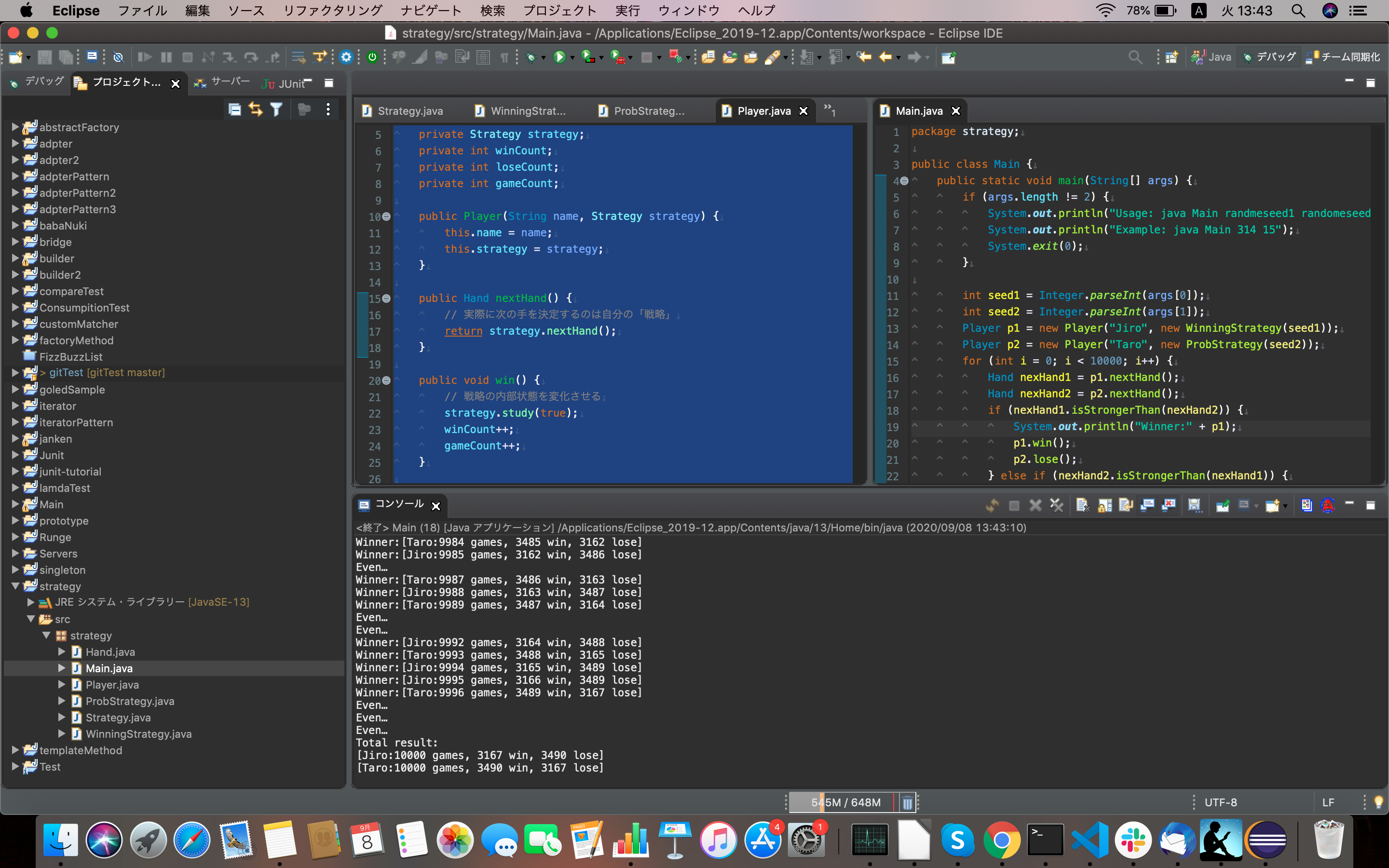# [JAVA] Strategy pattern

### What is the Strategy pattern?

Every program is written to solve a problem. And a specific algorithm is implemented to solve the problem. In the Strategy pattern, the part that implements the algorithm can be exchanged. A pattern that makes it easy to switch algorithms and solve the same problem differently.

### The role of Strategy

The role that defines the interface for using the strategy.

``````package strategy;

public interface Strategy {
//Decide what to do next
public abstract Hand nexHand();
//Learn if you won with the last hand
public abstract void study(boolean win);
}
``````

### The role of Concrete Strategy

The role that actually implements the interface of the Strategy role. Here, the strategy (strategy, method, policy, algorithm) is actually programmed.

``````package strategy;

import java.util.Random;

public class WinningStrategy implements Strategy{
private Random random;
private boolean won = false;
private Hand prevHand;

public WinningStrategy(int seed) {
random = new Random(seed);
}

/**
*If you win last time, you will get the same move, and if you lose, you will randomly decide your move.
*/
@Override
public Hand nextHand() {
if (!won) {
prevHand = Hand.getHand(random.nextInt(3));
}
return prevHand;
}

@Override
public void study(boolean win) {
won = win;
}
}
``````
``````package strategy;

import java.util.Random;

public class ProbStrategy implements Strategy {
private Random random;
private int prevHandValue = 0;
private int currentHandValue = 0;
//Table for probability calculation that reflects past wins and losses
//[The last hand][Hands to put out this time]
private int[][] history = {
{1,1,1,},
{1,1,1,},
{1,1,1,},
};

public ProbStrategy(int seed) {
random = new Random(seed);
}

/**
*Calculate with probability from the value of history
*For example, historyThe value of is 3, historyThe value of is 5, historyIf the value of is 7
*Goo, choki, par 3:5:Determine the next move as 7.
*0 or more and less than 15(15 is 3+5+Total value of 7)Get the random value of
*Goo if 0 or more and less than 3
*Choki if 3 or more and less than 8
*Par if 8 or more and less than 15
*To
*/
@Override
public Hand nextHand() {
int bet = random.nextInt(getSum(currentHandValue));
int handValue = 0;
if (bet < history[currentHandValue]) {
handValue = 0;
} else if (bet < history[currentHandValue] + history[currentHandValue]) {
handValue = 1;
} else {
handValue = 2;
}

prevHandValue = currentHandValue;
currentHandValue = handValue;
return Hand.getHand(handValue);
}

private int getSum(int hv) {
int sum = 0;
for (int i = 0; i < 3; i++) {
sum += history[hv][i];
}
return sum;
}

/**
*Update the contents of the history field based on the win or loss of the hand returned by the nextHand method
*
*/
@Override
public void study(boolean win) {
if (win) {
history[prevHandValue][currentHandValue]++;
} else {
history[prevHandValue][(currentHandValue + 1) % 3]++;
history[prevHandValue][(currentHandValue + 2) % 3]++;
}
}
}
``````

### The role of Context

A role that uses the Strategy role. I have an instance of the ConcreateStrategy role and use it as needed. It is the interface that plays the role of Strategy.

``````package strategy;

public class Player {
private String name;
private Strategy strategy;
private int winCount;
private int loseCount;
private int gameCount;

public Player(String name, Strategy strategy) {
this.name = name;
this.strategy = strategy;
}

public Hand nextHand() {
return strategy.nextHand();
}

public void win() {
//Change the internal state of the strategy
strategy.study(true);
winCount++;
gameCount++;
}

public void lose() {
//Change the internal state of the strategy
strategy.study(false);
loseCount++;
gameCount++;
}

public void even() {
gameCount++;
}

public String toString() {
return "[" + name + ":" + gameCount + " games, " + winCount + " win, " +
loseCount + " lose" + "]";
}
}
``````

### Caller

``````package strategy;

public class Main {
public static void main(String[] args) {
if (args.length != 2) {
System.out.println("Usage: java Main randmeseed1 randomeseed2");
System.out.println("Example: java Main 314 15");
System.exit(0);
}

int seed1 = Integer.parseInt(args);
int seed2 = Integer.parseInt(args);
Player p1 = new Player("Jiro", new WinningStrategy(seed1));
Player p2 = new Player("Taro", new ProbStrategy(seed2));
for (int i = 0; i < 10000; i++) {
Hand nexHand1 = p1.nextHand();
Hand nexHand2 = p2.nextHand();
if (nexHand1.isStrongerThan(nexHand2)) {
System.out.println("Winner:" + p1);
p1.win();
p2.lose();
} else if (nexHand2.isStrongerThan(nexHand1)) {
System.out.println("Winner:" + p2);
p1.lose();
p2.win();
} else {
System.out.println("Even…");
p1.even();
p2.even();
}
}

System.out.println("Total result:");
System.out.println(p1);
System.out.println(p2);

}
}
``````

### Execution resulthttps://github.com/aki0207/strategy

I used this as a reference. Augmented and Revised Introduction to Design Patterns Learned in Java Language